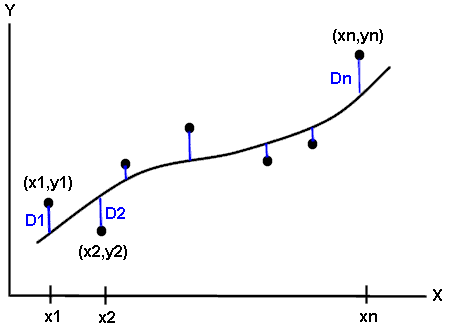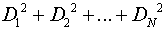Residuals and Least Squares

Developing a Model by Observation:
A simple type of regression equation is a straight line.  A scatter plot of the data is drawn, two points are chosen that "appear" to lie on the line of best fit, the slope is determined and an equation is written.  This is known as a freehand method of curve fitting.  Unfortunately, different observers, who choose different points, may obtain different equations.

Developing a Model by Least Squares:
To avoid individual judgment in curve fitting, it is necessary to agree on a definition of a “best-fitting line” or curve. Consider the following set of points:For a given value of x, say x1, there will be a difference between the value y1 and the corresponding value as determined by the “best fitting” curve. This distance, D1, is referred to as a residual.

A residual is the difference from the actual y-value and the value obtained by plugging the x-value (that goes with the y-value) into the regression equation.

Using these residuals, the following definition has been developed:

 Definition:  Of all curves approximating a given set of data points, the curve having the property thatis a minimum is called a best-fitting curve.

A curve having this property is said to fit the data in the least-squares sense and is called a least-squares curve.

The graphing calculator uses this least squares process to determine regression models.  When regression models are computed, residuals are automatically stored in a list called RESID.

Note:  For a perfect fit, the residuals will be all zero and ZOOM 9: ZoomStat will result in a WINDOW RANGE error since Ymin = 0 and Ymax = 0.  If you still wish to see the plot, change Ymin = -1 and Ymax = 1 and then press GRAPH.Finding Your Way Around TABLE of  CONTENTS